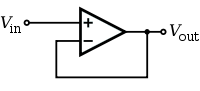# Buffer Opamp Amplifier

A unity gain buffer amplifier is implemented using an opamp in a negative feedback configuration. The output is connected to its inverting input, and the signal source is connected to the non-inverting input. Although its voltage gain is 1 or unity, it has high current gain, high input impedance and low output impedance. It is used to avoid loading of the signal source.The output voltage of the op-amp is given by the equation: \begin{equation} V_{out} = {A_{OL} \, (V_{\!+} - V_{\!-})} \end{equation} where V+ is the voltage at the non-inverting terminal, V− is the voltage at the inverting terminal and AOL is the open-loop gain of the amplifier.

Re-arrange the equation \begin{equation} {V_{out} \over A_{OL}} = V_{\!+} - V_{\!-} \end{equation}

If we assume that AOL is ∞, \begin{equation} V_- = V_+ \end{equation} For negative feedback opamp circuits only, we use equation 3 to simplify the analysis of the circuits.

For this buffer amplifier, using equation 3, we can by inspection obtain \begin{equation} V_{out} = V_- = V_+ = V_{in} \end{equation} and derive the gain of the buffer opamp amplifier \begin{equation} V_{out} = V_{in} \end{equation}

# Non Inverting Opamp Amplifier

The non inverting opamp amplifer provides voltage gain. The buffer amplifier can be considered as special case of this amplifer with Rf = 0 and Rg = ∞.If we let the input current to the inverting input I- = 0 and apply KVL to the Vout, Rf and Rg loop \begin{equation} V_- = {V_{out}} {{R_g }\over {R_f + R_g}} \end{equation} Applying equation 3, \begin{equation} V_{in} = V_+ = V_- = {V_{out}} {{R_g }\over {R_f + R_g}} \end{equation} Re-arrange the equation to obtain the gain of the non inverting opamp amplifer \begin{equation} V_{out} = (1 + {{R_f }\over {R_g}}) {V_{in}} \end{equation}

With advertising revenues falling despite increasing numbers of visitors, we need your help to maintain and improve this site, which takes time, money and hard work. Thanks to the generosity of our visitors who gave earlier, you are able to use this site at no charge.

If you have benefited from this site and are able, please give \$10 via Paypal. It will allow us continue into the future. It only takes a minute. Thanks!